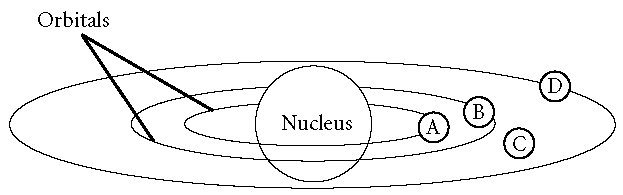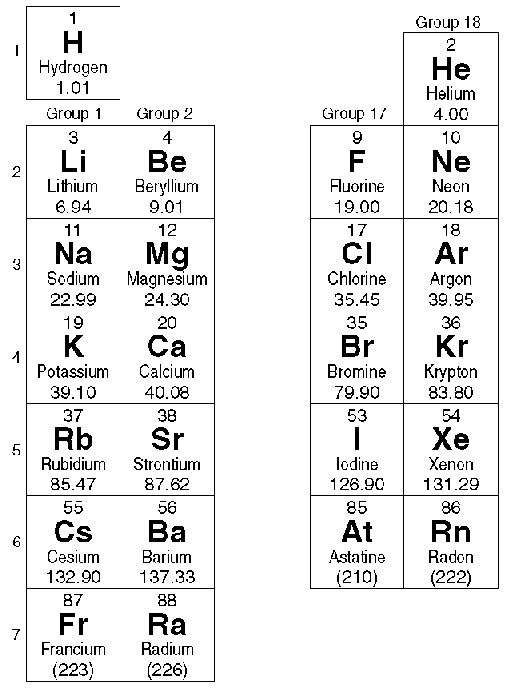# Quarter 1 exam Multiple Choice

 Page 1/2 Date conversion 26.11.2017 Size 240,59 Kb.
1   2
Quarter 1 exam

Multiple Choice

Identify the choice that best completes the statement or answers the question.

____ 1. If two or more compounds are composed of the same two elements, the ratio of the masses of one element that combine with a fixed mass of the other element is a simple whole number. This is a statement of the law of

 a. conservation of mass. c. multiple proportions. b. mass action. d. definite composition.

____ 2. Oxygen can combine with carbon to form two compounds, carbon monoxide and carbon dioxide. The ratio of the masses of oxygen that combine with a given mass of carbon is 1:2. This is an example of

 a. the law of conservation of mass. c. the law of conservation of energy. b. Dalton's atomic theory. d. the law of multiple proportions.

____ 3. The law of definite proportions

 a. contradicted Dalton's atomic theory. b. agrees with Dalton's atomic theory. c. replaced the law of conservation of mass. d. assumes that atoms of all elements are identical.

____ 4. Dalton's atomic theory agrees with modern atomic theory except for the statement that

 a. all matter is made up of small particles. b. atoms are not divided in chemical reactions. c. atoms of the same element are chemically alike. d. all atoms of the same element have the same mass.

____ 5. The atomic theory proposed by Dalton has been

 a. totally discarded. c. accepted unchanged to the present day. b. expanded and modified. d. found to be plagiarized.

____ 6. Experiments with cathode rays led to the discovery of the

 a. proton. c. neutron. b. nucleus. d. electron.

____ 7. After measuring the ratio of the charge of a cathode-ray particle to its mass, Thomson concluded that the particles

 a. had no mass. c. had a very large mass. b. had a very small mass. d. carried a positive charge.

____ 8. Whose series of experiments identified the nucleus of the atom?

 a. Rutherford c. Chadwick b. Dalton d. Bohr

____ 9. Because most particles fired at metal foil passed straight through, Rutherford concluded that

 a. atoms were mostly empty space. c. electrons formed the nucleus. b. atoms contained no charged particles. d. atoms were indivisible.

____ 10. Rutherford's experiments led him to conclude that atoms contain massive central regions that have

 a. a positive charge. c. no charge. b. a negative charge. d. both protons and electrons.

____ 11. A nuclear particle that has about the same mass as a proton, but with no electrical charge, is called a(n)

 a. nuclide. c. electron. b. neutron. d. isotope.

____ 12. The smallest unit of an element that can exist either alone or in combination with other such particles of the same or different elements is the

 a. electron. c. neutron. b. proton. d. atom.

____ 13. Isotopes are atoms of the same element that have different

 a. principal chemical properties. c. numbers of protons. b. masses. d. numbers of electrons.

____ 14. The most common form of hydrogen has

 a. no neutrons. c. two neutrons. b. one neutron. d. three neutrons.

____ 15. The total number of protons and neutrons in the nucleus of an atom is its

 a. atomic number. c. mass number. b. Avogadro number. d. average atomic mass.

____ 16. As the atomic number increases, the number of electrons in a neutral atom

 a. decreases. c. remains the same. b. increases. d. is undetermined.

____ 17. All atoms of the same element have the same

 a. atomic mass. c. mass number. b. number of neutrons. d. atomic number.

____ 18. The atomic mass of an element listed in the periodic table is the

 a. average atomic mass. b. relative atomic mass of the most abundant isotope. c. relative atomic mass of the most abundant radioactive isotope. d. mass number of the least abundant isotope.

____ 19. Chlorine has atomic number 17 and mass number 35. It has

 a. 17 protons, 17 electrons, and 18 neutrons. b. 35 protons, 35 electrons, and 17 neutrons. c. 17 protons, 17 electrons, and 52 neutrons. d. 18 protons, 18 electrons, and 17 neutrons.

____ 20. Avogadro's number is

 a. the maximum number of electrons that all the energy levels can accommodate. b. the number of protons and neutrons that can fit in the shells of the nucleus. c. the number of particles in 1 mole of a pure substance. d. the number of particles in exactly 1 gram of a pure substance.

____ 21. Molar mass

 a. is the mass in grams of one mole of a substance. b. is numerically equal to the average atomic mass of the element. c. Both (a) and (b) d. Neither (a) nor (b)

____ 22. The mass of 5.000 mol of cesium (atomic mass 132.9 amu) is

 a. 664.5 g. c. 6.022 ´ 1023 g. b. 132.9 g. d. 5.000 g.

____ 23. Max Planck proposed that a hot object radiated energy in small, specific amounts called

 a. quanta. c. hertz. b. waves. d. electrons.

____ 24. Bohr's theory helped explain why

 a. electrons have negative charge. b. most of the mass of the atom is in the nucleus. c. excited hydrogen gas gives off certain colors of light. d. atoms combine to form molecules.

____ 25. According to Bohr, electrons cannot reside at ____ in the figure below.a. point A c. point C b. point B d. point D

____ 26. Louis de Broglie's research suggested that

 a. frequencies of electron waves do not correspond to specific energies. b. electrons usually behave like particles and rarely like waves. c. electrons should be considered as waves confined to the space around an atomic nucleus. d. electron waves exist at random frequencies.

____ 27. The region outside the nucleus where an electron can most probably be found is the

 a. electron configuration. c. s sublevel. b. quantum. d. electron cloud.

____ 28. The size and shape of an electron cloud are most closely related to the electron's

 a. charge. c. spin. b. mass. d. energy.

____ 29. The set of orbitals that are dumbbell shaped and directed along the x, y, and z axes are called

 a. d orbitals. c. f orbitals. b. p orbitals. d. s orbitals.

____ 30. The major difference between a 1s orbital and a 2s orbital is that

 a. the 2s orbital can hold more electrons. b. the 2s orbital has a slightly different shape. c. the 2s orbital is at a higher energy level. d. the 1s orbital can have only one electron.

____ 31. The number of orbitals for the d sublevel is

 a. 1 c. 5 b. 3 d. 7

____ 32. How many electrons can occupy the s orbitals at each energy level?

 a. two, if they have opposite spins b. two, if they have the same spin c. one d. no more than eight

____ 33. The Pauli exclusion principle states that no two electrons in the same atom can

 a. occupy the same orbital. b. have the same spin quantum numbers. c. have the same set of quantum numbers. d. be at the same main energy level.

____ 34. What is the electron configuration for nitrogen, atomic number 7?

 a. 1s2 2s2 2p3 b. 1s2 2s3 2p2 c. 1s2 2s3 2p1 d. 1s2 2s2 2p2 3s1

____ 35. The idea of arranging the elements in the periodic table according to their chemical and physical properties is attributed to

 a. Mendeleev. c. Bohr. b. Moseley. d. Ramsay.

____ 36. Mendeleev predicted that the spaces in his periodic table represented

 a. isotopes. c. unstable elements. b. radioactive elements. d. undiscovered elements.

____ 37. The periodic law allows some properties of an element to be predicted based on its

 a. position in the periodic table. c. symbol. b. number of isotopes. d. color.

____ 38. The atomic number of lithium, the first element in Group 1, is 3. The atomic number of the second element in this group is

 a. 4 c. 11 b. 10 d. 18

____ 39. Refer to the figure below. To which group do fluorine and chlorine belong?a. alkaline-earth metals c. halogens b. transition elements d. actinides

____ 40. Hydrogen is placed separately from other elements in the periodic table because it

 a. is a gas. b. does not exist as a free element in nature. c. has atomic number one. d. has many unique properties.

____ 41. To which block do the actinide elements belong?

 a. d block c. f block b. s block d. p block

____ 42. For groups 13 through 18, the total number of electrons in the highest occupied level equals the group number

 a. plus 1. c. plus 5. b. minus 1. d. minus 10.

____ 43. Bromine, atomic number 35, belongs to Group 17. How many electrons does bromine have in its outermost energy level?

 a. 7 c. 18 b. 17 d. 35

____ 44. The most reactive group of the nonmetals is the

 a. lanthanides. c. halogens. b. transition elements. d. noble gases.

____ 45. The first member of the noble gas family, whose highest energy level consists of an octet of electrons, is

 a. helium. c. neon. b. argon. d. krypton.

____ 46. The most characteristic property of the noble gases is that they

 a. have low boiling points. b. are radioactive. c. are gases at ordinary temperatures. d. are largely unreactive.

____ 47. Compared to the alkali metals, the alkaline-earth metals

 a. are less reactive. b. have lower melting points. c. are less dense. d. combine more readily with nonmetals.

____ 48. A measure of the ability of an atom in a chemical compound to attract electrons from another atom in the compound is called

 a. electron affinity. c. electronegativity. b. electron configuration. d. ionization potential.

____ 49. One-half the distance between the nuclei of identical atoms that are bonded together is called the

 a. atomic radius. c. atomic volume. b. atomic diameter. d. electron cloud.

____ 50. A positive ion is known as a(n)

 a. ionic radius. c. cation. b. valence electron. d. anion

____ 51. In a row in the periodic table, as the atomic number increases, the atomic radius generally

 a. decreases. c. increases. b. remains constant. d. becomes immeasurable.

____ 52. The number of valence electrons in Group 1 elements is

 a. 1 c. 8. b. 2 d. equal to the period number.

____ 53. For groups 13 through 18, the number of valence electrons is equal to the group number

 a. plus 1. c. minus the period number. b. plus the period number. d. minus 10.

1   2

The database is protected by copyright ©sckool.org 2016
send message

Main page